# Natural numbers - 6th grade (11y) - math problems

#### Number of problems found: 220

• Intersect of setsRefer to the sets below: U = {1,2,3,. .. ,10} A = {1,2,3,4,5} B = {2,4,6,7,8} C = {4,5,6,8,10} A ∩ B ∩ C = ___
• How many 17How many fifths are there in four wholes? How many 1/5 are there in 4?
• 30 eggs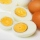There are 30 eggs in a tray. If 1/2 of the tray used 1/5 of it cooked,1/3 kept the refrigerator, how many eggs were left?
• Athletics teamAll athletes in the athletics team from Ostrava can start in four, five, six and seven stages, and no one will be present. How many average athletes are in one athletic group if there are a total of twelve groups in the section? Consider the smallest numb
• Simplest form 3What fraction is 15 of 35 in simplest form?
• English and math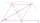1/5 of a class study English, and 3/5 study mathematics. If the class consist of 80 students, how many students study English and mathematics in the class?
• SixteenSixteen minus the quotient of 12 and what number is thirteen?
• Four multiplesFour multiples of 6 he writes the following 12  24  56  72, which is correct?
• The cost 2The cost of 5 apples is \$3.45, and 5 oranges are \$1.23. If Rachel buys one apple and one orange, then how much must she pay?
• The buildingThere are 45 people living in building, out of these 3/5 are men,2/5 are women . find the number of men and women in the building.
• Players - baseball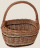There are 20 players on each of two baseball teams. If 2/5 of the players on team 1 miss practice and 1/4 of the players on team two miss practice, how many more players from team 1 missed practice then team 2?
• What fraction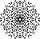What fraction of number 1 to 30 is prime?
• A teacher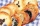A teacher baked 5 dozen cookies for the 16 students in her class. If the teacher divided the cookies equally among her students, how many cookies did she give each student?
• There 14There are 250 people in a museum. 2/5 of the 250 people are girls 3/10 of the 250 people are boys The rest of the 250 people are adults Work out the number of adults in the museum.
• Evaluate 12Evaluate and simplify 9 divided by 7/6.The local reader’s club has a set of 28 hardback books and a set of 44 paperbacks. Each set can be divided equally among the club members. What is the greatest possible number of club members?
• Monica 3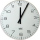Monica works on her drawings for 2 hours. She spends 1/6 hour on each drawing. How many drawings does she work on in 2 hours?
• Evaluate 11Multiply the quotient of 6 and 2 by 3, then add 1. Add 1 and 6, then divide by 2 and multiply by 3. Divide 6 by the product of 2 and 3, then add 1.
• 5 2/55 2/5 hours a week  mathematics,  3 3/4 hours a week   Natural sciences, 4 3/8 hours a week  Technology . how many hours does  he spend on social sciences if he spend 17 1/2 hours a week for the four subject?
• Each year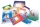Each year, Mr. Baumann purchases enough paper for his 16 students. If each student will use 3/4 pack of paper, how many packs of paper will he need to purchase? Answer as a mixed number or as a whole number.

Do you have an exciting math question or word problem that you can't solve? Ask a question or post a math problem, and we can try to solve it.

We will send a solution to your e-mail address. Solved examples are also published here. Please enter the e-mail correctly and check whether you don't have a full mailbox.

Natural numbers - math problems. Examples for 6th grade (the sixth graders).